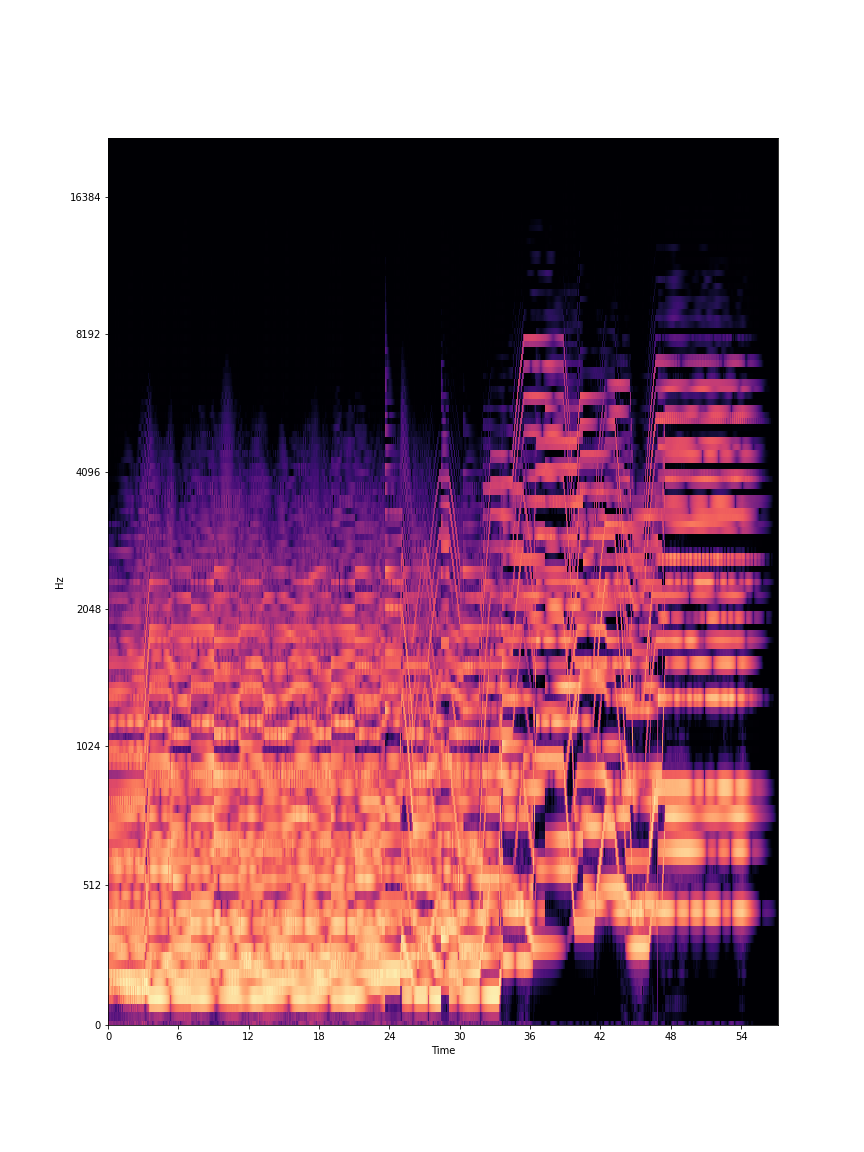# MFCC

Mel-Frequency Cepstrum Coefficients

MFCC stands for Mel-Frequency Cepstral Coefficients (“cepstral” is pronounced like “kepstral”). This analysis is often used for timbral description and timbral comparison. It compresses the overall spectrum into a smaller number of coefficients that, when taken together, describe the general contour of the spectrum.

The MFCC values are derived by first computing a mel-frequency spectrum, just as in MelBands. `numCoeffs` coefficients are then calculated by using that mel-frequency spectrum as input to the discrete cosine transform. This means that the shape of the mel-frequency spectrum is compared to a number of cosine wave shapes (different cosines shapes created from different frequencies). Each MFCC value (i.e., “coefficient”) represents how similar the mel-frequency spectrum is to one of these cosine shapes.

## MFCCs as a Form of Data Compression

In the demo below, use the slider to adjust the number of coefficients (MFCCs) used to represent the spectrum. With more coefficients, the spectrum (orange line) is more accurately represented by the blue line. With fewer coefficients, the blue line looses precision in representing the spectrum, but still reflects the general spectral contour. This demonstrates why using a lower number of coefficients can still provide relevant information about the contour of the spectrum.

Note that the blue line seen on the right is not the actual coefficients of the MFCC. Instead it is an inverse discrete cosine transform of the cepstrum used here for display purposes. The blurriness of the spectrogram on the left mirrors the lower resolution spectral representation that MFCCs offer.

127 MFCCsPointer

Other than the 0th coefficient, MFCCs are unchanged by differences in the overall energy of the spectrum (which relates to how we perceive loudness). This means that timbres with similar spectral contours, but different volumes, will still have similar MFCC values, other than MFCC 0. To remove any indication of loudness but keep the information about timbre, we can ignore MFCC 0 by setting the parameter `startCoeff` = 1.